﻿ 魔方入门解法第7步：调整顶层棱块位置 - 爱魔方 ﻿

## 7. 调整顶层棱块位置，还原魔方

目标：调整好顶层棱块位置，如下图所示：

 完成前 完成后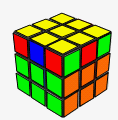→转动窍门：

详细图解：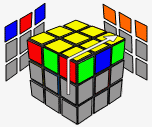→→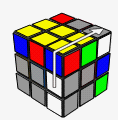→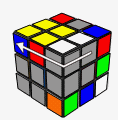R U' R U →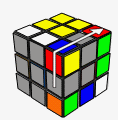→→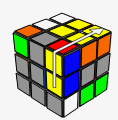→R U R U' →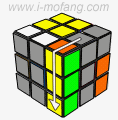→→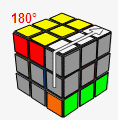→R' U' R2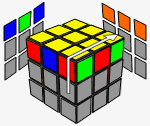→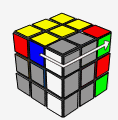→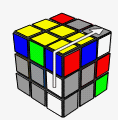→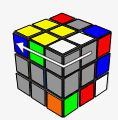R U' R U →→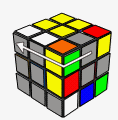→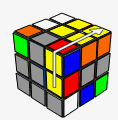→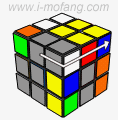R U R U' →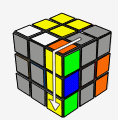→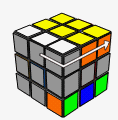→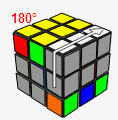→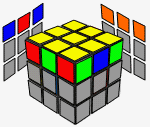R' U' R2 情况1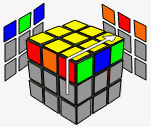→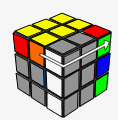→→R U' R U →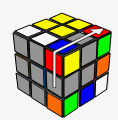→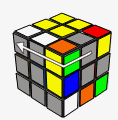→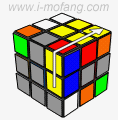→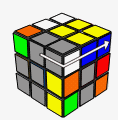R U R U' →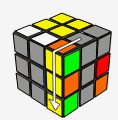→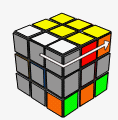→→R' U' R2 向左转一下顶层 →情况1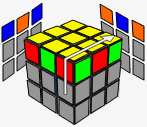→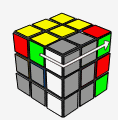→→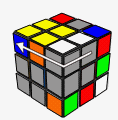R U' R U →→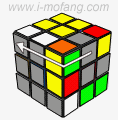→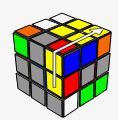→R U R U' →→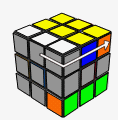→→R' U' R2 向右转一下顶层 →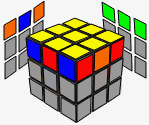情况1

## ◆